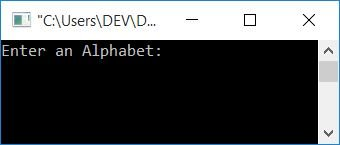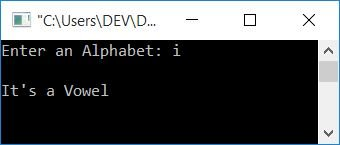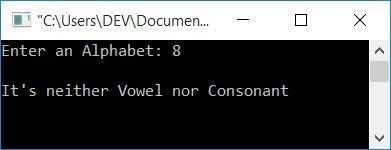# C Program to Check Vowel or Consonant

In this article, you will learn how to check whether the character given by the user (at runtime) is a vowel or a consonant. But before we get started, let's understand what a vowel and a consonant are.

### Vowels and consonants

There are 5 characters (a, e, i, o, u) from all the 26 characters of the alphabet that are known as vowels. Vowels in alphabetical order:

• a
• e
• i
• o
• u
• A
• E
• I
• O
• U

The first five characters are lowercase vowels, and the second five characters are uppercase vowels. Except for vowels, all the other 21 characters are known as consonants.

## In C, check for a vowel or a consonant

To check whether the input alphabet is a vowel or consonant in C programming, you have to ask the user to enter a character and check if the given character is equal to a, A, e, E, i, I, o, O, u, U, or not. If it is equal to any one of these 10, then it is a vowel; otherwise, it is a consonant. Let's have a look at the program:

```#include<stdio.h>
#include<conio.h>
int main()
{
char ch;
printf("Enter an Alphabet: ");
scanf("%c", &ch);
if(ch=='a' || ch=='e' || ch=='i' || ch=='o' || ch=='u')
printf("\nIt's a Vowel");
else if(ch=='A' || ch=='E' || ch=='I' || ch=='O' || ch=='U')
printf("\nIt's a Vowel");
else
printf("\nIt's a Consonant");
getch();
return 0;
}```

This program is compiled and executed in the Code::Blocks IDE. The snapshot given below shows the sample run above of this program:Now supply any alphabet (A-Z or a-z), say "i," and press the ENTER key to see the output as shown in the snapshot given below:Because i is a vowel in the alphabet, so you have seen the above output. The previous program is true only when the user supplies any input that comes under the alphabet.But what if user enters any input as a special character, say #?
because # is neither equal to a lowercase vowel (a, e, i, o, u) nor equal to an uppercase vowel (A, E, I, O, U).

As a result, the else block gets executed, the program will print the output It's a Consonant. And we all knows that # is a special character, not a consonant. Therefore, let's modify this program to match the one given below.

```#include<stdio.h>
#include<conio.h>
int main()
{
char ch;
printf("Enter an Alphabet: ");
scanf("%c", &ch);
if((ch>='a' && ch<='z') || (ch>='A' && ch<='Z'))
{
if(ch=='a' || ch=='e' || ch=='i' || ch=='o' || ch=='u')
printf("\nIt's a Vowel");
else if(ch=='A' || ch=='E' || ch=='I' || ch=='O' || ch=='U')
printf("\nIt's a Vowel");
else
printf("\nIt's a Consonant");
}
else
printf("\nIt's neither Vowel nor Consonant");
getch();
return 0;
}```

If you enter any letter, then this program will produce the same output as the first program given in this article. But if the user enters any character other than an alphabet, say 5, then this program will produce the output as given below:In the above program, before checking for a vowel or consonant, We have applied an extra code that checks whether the given character falls under a-z or A-Z or not. If it is an alphabet, then it will continue to check for a vowel or consonant; otherwise, the outer else block gets executed, and It's neither Vowel nor Consonant gets printed on the output screen as shown in the sample run given above.

## Check if the given alphabet is a vowel

It's a simple program that only checks for vowels. That is, if the user enters a vowel character, then this program will print it as a vowel; otherwise, It's not a Vowel gets printed.

```#include<stdio.h>
#include<conio.h>
int main()
{
char ch;
printf("Enter an Alphabet: ");
scanf("%c", &ch);
if(ch=='a' || ch=='e' || ch=='i' || ch=='o' || ch=='u')
printf("\nIt's a Vowel");
else if(ch=='A' || ch=='E' || ch=='I' || ch=='O' || ch=='U')
printf("\nIt's a Vowel");
else
printf("\nIt's not a Vowel");
getch();
return 0;
}```

You can also create another program that performs the same functions as the one described above. Here is the program:

```#include<stdio.h>
#include<conio.h>
int main()
{
char ch;
int lowerVowel, upperVowel;
printf("Enter an Alphabet: ");
scanf("%c", &ch);
lowerVowel = (ch=='a' || ch=='e' || ch=='i' || ch=='o' || ch=='u');
upperVowel = (ch=='A' || ch=='E' || ch=='I' || ch=='O' || ch=='U');
if(lowerVowel || upperVowel)
printf("\nIt's a Vowel");
else
printf("\nIt's not a Vowel");
getch();
return 0;
}```

Let's go over some of the steps in the preceding program:

• Scan a character input into the ch variable.
• If ch is equal to any of the lowercase vowels (a, e, i, o, u). Then 1 is set to lowerVowel.
• Or if ch is equal to any of the uppercase vowels (A, E, I, O, U). Then again, 1 is set to upperVowel.
• Now use the if-else case to check whether lowerVowel or upperVowel contains 1 or not.
• If either of the two variables, lowerVowel and upperVowel, contains a 1, the condition of the if block evaluates to true, and It's a Vowel gets printed.
• Otherwise, if both variables, say lowerVowel and upperVowel, contains 0. And because 0 is considered false, the condition of the if block evaluates to false, and the else block is executed, and It's not a Vowel gets printed on the output console.

#### The same program in different languages

C Quiz

« Previous Program Next Program »